# rfsystem

Generate RF Blockset model and perform circuit envelope or idealized baseband simulation

Since R2021a

## Description

Use the `rfsystem` System object™ to generate RF Blockset™ model and perform circuit envelope and idealized baseband (since R2023a) simulation of an RF system designed using an `rfbudget` object. This object supports vector inputs and has no limitations on the frame size.

Note

You can add or delete RF Blockset blocks from the model but you cannot modify the parameters of the Inport and Outport blocks. After this update, the input `rfbudget` object to the `rfsystem` will be preserved and you can inspect this `rfbudget` object using the RF Budget Analyzer app.

To perform circuit envelope simulation of an RF system:

1. Create the `rfsystem` object and set its properties.

2. Call the object with arguments, as if it were a function.

## Creation

### Syntax

``rfs = rfsystem(rfb)``
``rfs = rfsystem(rfb,Name=Value)``

### Description

example

````rfs = rfsystem(rfb)` generates an RF Blockset model from the RF budget object `rfb` and perform circuit envelope or idealized baseband (since R2023a) simulation. Use Object Functions to open, save, close, or hide the RF Blockset model.```

example

````rfs = rfsystem(rfb,Name=Value)` sets Properties using name-value arguments. For example, `rfsystem(rfb,'ModelName'='rfmodel')` sets the name of the RF Blockset model to `rfmodel`. ```

## Properties

expand all

Unless otherwise indicated, properties are nontunable, which means you cannot change their values after calling the object. Objects lock when you call them, and the `release` function unlocks them.

If a property is tunable, you can change its value at any time.

Name of the RF Blockset model, specified as a string scalar or character vector.

Example: `'ModelName','RFModel'`

Input frequency for each input in the RF system, specified as a row vector in Hz. The default value of this property is the value you specify in the `InputFrequency ` argument in the `rfbudget` object.

If the `rfb.InputFrequency(1)` is set to `0`, then in:

• Circuit Envelope Simulation — Your RF system has two inputs, I and Q, at DC.

• Idealized Baseband Simulation — Your RF system input is at DC with the I and Q components distributed on the real and imaginary parts of the signal input argument. (since R2023a)

Note

You can set `InputFrequency` to a vector after creating the System object if you want to investigate the intermodulation of nearby signals using the same model.

Output frequency for each output of the RF system, specified as a row vector in Hz. This output frequency is derived from the RF system designed using an `rfbudget` object.

If the value of the `rfb.OutputFrequency(1)` is `0`, then in:

• Circuit Envelope Simulation — Your RF system will have two outputs, I and Q, at DC .

• Idealized Baseband Simulation — Your RF system output is at DC, with the I and Q components distributed on the real and imaginary components of the signal returned output. (since R2023a)

Time step for circuit envelope simulation, specified as a positive scalar. This property sets the step size between simulations.

Number of input chains in a multiple-input single-output receiver system, specified as a positive scalar.

Note

You can set:

• Either the `'Rx'` or the `'Tx'` property when creating the System object.

• `'Rx'` property only when creating the System object.

Number of output chains in a single-input multiple-output transmitter system, specified as a positive scalar.

Note

You can set:

• Either the `'Rx'` or the `'Tx'` property when creating the System object.

• `'Rx'` property only when creating the System object.

By default, the parameter in the Simulink Inport blocks is set to `complex`. To change this complex signal to real, either add a Complex to Real-Imag (Simulink) block to your model, or in the Simulink Inport block, on the tab set the parameter `real`.

By default, the parameter in the Simulink Outport block is set to `complex`. To change this complex signal to real, either add a Complex to Real-Imag (Simulink) block to your model, or in the Simulink Inport block, on the tab set the parameter `real`.

Number of RF inputs implemented as RF Blockset Inport blocks in the generated model, returned as a nonnegative scalar. The value of this property depends on the 'Rx' property and the first value in the 'InputFrequency' row vector.

Number of RF outputs implemented as RF Blockset Outport blocks in the generated model, returned as a nonnegative scalar. The value of this property depends on the 'Tx' property and the last value in the 'OutputFrequency' row vector.

Since R2023a

RF Blockset libraries, specified as one of the following:

• `'CircuitEnvelope'` — Use Circuit Envelope library blocks to perform circuit envelope simulation.

• `'IdealizedBaseband'` — Use Idealized Baseband library blocks to perform idealized baseband simulation.

## Usage

### Syntax

``out = rfs(in)``

### Description

example

````out = rfs(in)` creates an RF Blockset circuit envelope or idealized baseband simulation output `out` using input signal values `in`. Pass `in` as an input argument to an automatically-generated RF Blockset model. You can design four architectures, RF to RF, DC to RF, RF to DC, and DC to DC, using the `rfsystem` object. For more information, see Design RF-RF, IQ-RF, RF-IQ, and IQ-IQ Architectures. NotePassing multiple input vectors and concatenating the output vectors is equivalent to performing one long simulation with a vertically-concatenated input. ```

### Input Arguments

expand all

Time-domain input signal, specified as a scalar or column vectors.

• Circuit Envelope Simulation — Specify a column vector of N columns, where N is the number of input frequencies in the 'InputFrequency' property.

• Idealized Baseband Simulation — Specify a scalar because idealized Baseband library only supports one carrier frequency. (since R2023a)

If 'RFInputs' > `1` , 'SLInputs' > `1`, or both, pass the inputs to the `step` function as arguments ```out = rfs(RFin1,RFin2,...,SLin1,SLin2,...)```.

### Output Arguments

expand all

Time-domain output signal, returned as scalar or column vectors. The `out` is returned as an array of N column vectors when there are N 'OutputFrequency' values to be computed.

If 'RFOutputs' > `1`, 'SLOutputs' > `1`,or both, then the outputs are returned by the `step` function is ```[RFout1,RFout2,...,SLout1,SLout2,...] = rfs(in)```.

## Object Functions

To use an object function, specify the System object™ as the first input argument. For example, to release system resources of a System object named `obj`, use this syntax:

`release(obj)`

expand all

 `open_system` Open RF Blockset model created using `rfsystem` `save_system` Save RF Blockset model created using `rfsystem` `close_system` Close RF Blockset model window created using `rfsystem` `hide_system` Hide RF Blockset model window created using `rfsystem` `load_system` Load RF Blockset model to memory RF Budget Analyzer Analyze gain, noise figure, IP2, and IP3 of cascaded RF elements and export to RF Blockset

expand all

 `step` Run System object algorithm `release` Release resources and allow changes to System object property values and input characteristics `reset` Reset internal states of System object

## Examples

collapse all

Design an RF receiver to perform circuit envelope simulation.

Create fifth- and seventh-order bandpass RF filters.

```f1 = rffilter('ResponseType','Bandpass','FilterOrder',5, ... 'PassbandFrequency',[4.85 5.15]*1e9); f2 = rffilter('ResponseType','Bandpass','FilterOrder',7, ... 'PassbandFrequency',[10 130]*1e6); ```

Create two amplifier objects with `3` dB and `5` dB gain, respectively.

```a1 = amplifier('Gain',3,'NF',1.53,'OIP3',35); a2 = amplifier('Gain',5,'NF',8,'OIP3',37); ```

Create a modulator with a local frequency of `4.93` GHz.

```d = modulator('Gain',0,'NF',4,'OIP3',50,'LO',4.93e9, ... 'ConverterType','Down'); ```

Design an RF receiver with the budget elements at an input frequency of `5` GHz, an available input power of `-30` dBm, and a bandwidth of `200` MHz.

```rfb = rfbudget([f1 a1 d f2 a2],5e9,-30,200e6); ```

Create an RF system for the RF receiver using the `rfbudget` object.

```rfs = rfsystem(rfb); ```

Specify input time-domain signal for the RF system.

```in = [1e-3*ones(8,1); zeros(8,1)] .* ones(1,10); in = in(:); ```

Calculate the output time-domain signal of the RF system.

```out = rfs(in); out = [out; rfs(in)]; ```

Specify the sample time of the RF system.

```t = rfs.SampleTime*(0:length(out)-1); ```

Plot the simulated output.

```plot(t,[in; in],'-o',t,abs(out),'-+') grid on ```Release system resources and turn off fast restart.

```release(rfs) ```

Open an RF Blockset model of the designed RF system using the `open_system` object function.

```open_system(rfs) ```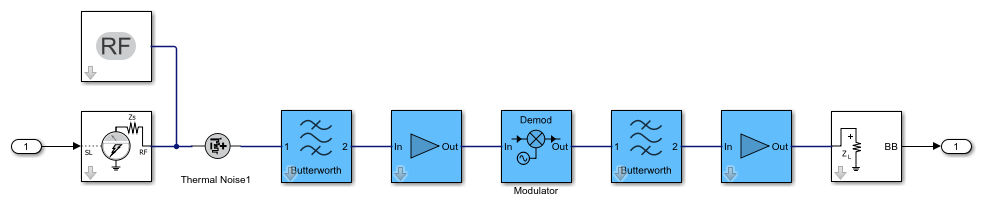Design four different chain architectures using an RF System object.

Create an input column vector.

```in = (1:8)'; ```

Design RF-RF Architecture

Create an `rfbudget` object using an `amplifier` object.

```a = amplifier; ```

Calculate the RF budget of the amplifier at an input frequency of `5` GHz, an available input power of `–30` dBm, and a bandwidth of `10` KHz.

```rfb = rfbudget(a,5e9,-30,10e3); ```

Create an RF system using the `rfbudget` object.

```rfs = rfsystem(rfb); ```

Create an RF-RF architecture using the input column vector.

```out0 = rfs(in); ```

Release system resources and turn off fast restart.

```release(rfs) ```

Open an RF Blockset model of the RF system.

```open_system(rfs) ```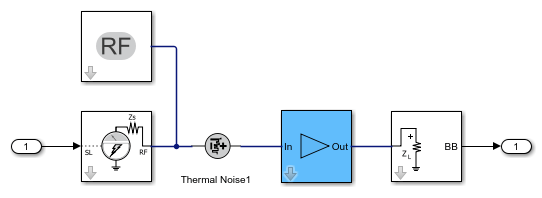Design IQ-RF Architecture

Use a `modulator` object with an up converter to create an `rfbudget` object.

```u = modulator('ConverterType','Up','LO',1e9); ```

Calculate the RF budget of the modulator at an input frequency of `0` GHz, an available input power of `–30` dBm, and a bandwidth of `10` KHz.

```rfb2 = rfbudget(u,0,-30,10e3); ```

Create an RF system using the `rfbudget` object.

```rfs2 = rfsystem(rfb2); ```

Create an IQ-RF architecture using the input column vector.

```inI = in; inQ = in; out = rfs2(inI,inQ); ```

Release system resources and turn off fast restart.

```release(rfs2) ```

Open an RF Blockset model of the RF system.

```open_system(rfs2) ```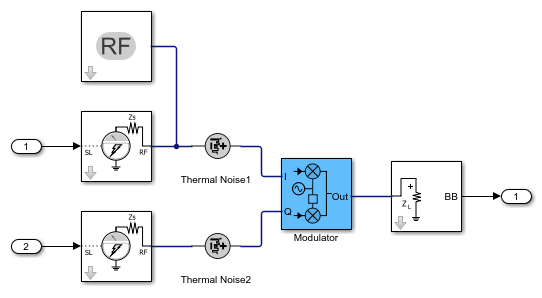Design RF-IQ Architecture

Use a `modulator` object with a down converter to create an `rfbudget` object.

```d = modulator('ConverterType','Down','LO',1e9); ```

Calculate the RF budget of the modulator at an input frequency of `1` GHz, an available input power of `–30` dBm, and a bandwidth of `10` KHz.

```rfb3 = rfbudget(d,1e9,-30,10e3); ```

Create an RF system using the `rfbudget` object.

```rfs3 = rfsystem(rfb3); ```

Create an RF-IQ architecture using the input column vector.

```[outI,outQ] = rfs3(in); ```

Release system resources and turn off fast restart.

```release(rfs3) ```

Open an RF Blockset model of the RF system.

```open_system(rfs3) ```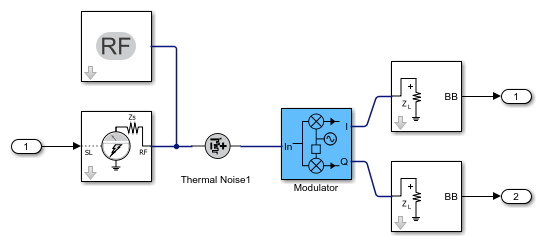Design IQ-IQ Architecture

Create an `rfbudget` object using an `amplifier` object.

```a1 = amplifier; ```

Calculate the RF budget of the amplifier at an input frequency of `0` GHz, an available input power of `–30` dBm, and a bandwidth of `10` KHz.

```rfb4 = rfbudget(a1,0,-30,10e3); ```

Create an RF system using the `rfbudget` object.

```rfs4 = rfsystem(rfb4); ```

Create an IQ-IQ architecture using the input column vector.

```[outI2,outQ2] = rfs4(inI,inQ); ```

Release system resources and turn off fast restart.

```release(rfs4) ```

Open an RF Blockset model of the RF system.

```open_system(rfs4) ```Create a fifth-order bandpass RF filter.

```f1 = rffilter('ResponseType','Bandpass','FilterOrder',5, ... 'PassbandFrequency',[4.85 5.15]*1e9); ```

Create an amplifier with 3 dB gain.

```a1 = amplifier('Gain',3,'NF',1.53,'OIP3',35); ```

Create a modulator with a local frequency of 4.93 GHz.

```d = modulator('Gain',0,'NF',4,'OIP3',50,'LO',4.93e9, ... 'ConverterType','Down'); ```

Create a seventh-order bandpass RF filter.

```f2 = rffilter('ResponseType','Bandpass','FilterOrder',7, ... 'PassbandFrequency',[10 130]*1e6); ```

Create another amplifier with a 3 dB gain.

```a2 = amplifier('Gain',5,'NF',8,'OIP3',37); ```

Design an RF receiver with the budget elements at an input frequency of 5 GHz, an available input power of –30 dBm, and a bandwidth of 200 MHz.

```b = rfbudget([f1 a1 d f2 a2],5e9,-30,200e6); ```

Duplicate the budget chain into a multiple-input single-output receiver (MISO) system with four branches.

```rfs = rfsystem(b,Rx=4); ```

Open the underlying RF system model to inspect the MISO receiver

```open_system(rfs) ``````set_param(bdroot,'ZoomFactor','FitSystem') ```

Create four inputs to the MISO receiver. The pulsed carrier square wave input is time-sliced into four pieces.

```in1 = [1e-3*[1;1;0;0;0;0;0;0]; zeros(8,1)] .* ones(1,10); in1 = in1(:); in2 = [1e-3*[0;0;1;1;0;0;0;0]; zeros(8,1)] .* ones(1,10); in2 = in2(:); in3 = [1e-3*[0;0;0;0;1;1;0;0]; zeros(8,1)] .* ones(1,10); in3 = in3(:); in4 = [1e-3*[0;0;0;0;0;0;1;1]; zeros(8,1)] .* ones(1,10); in4 = in4(:); out = rfs(in1,in2,in3,in4); ```

Release system resources and turn off fast restart.

```release(rfs) reset(rfs) ```

Plot the circuit envelope simulated result.

```t = rfs.SampleTime*(0:length(out)-1); plot(t,in1+in2+in3+in4,'-o',t,abs(out),'-+') grid on ```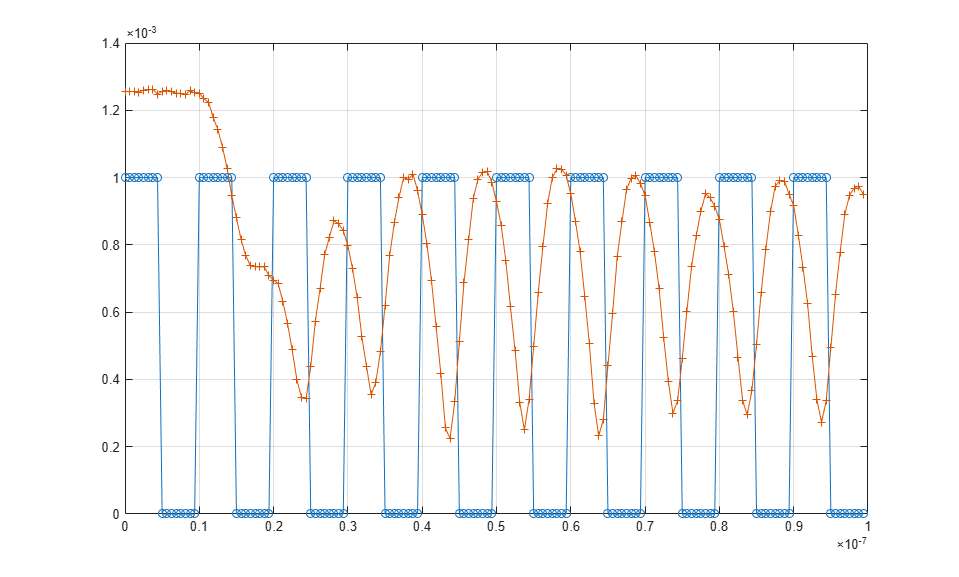Type `rfBudgetAnalyzer(rfs)` command at the command line to open the MISO receiver in the RF Budget Analyzer app to visualize the initial budget chain `b`.Duplicate the budget chain into a single-input multiple-output (SIMO) array system with sixteen branches.

```rfs2 = rfsystem(b,Tx=16); ```

Open the underlying RF system model to inspect the SIMO receiver

```open_system(rfs2) ```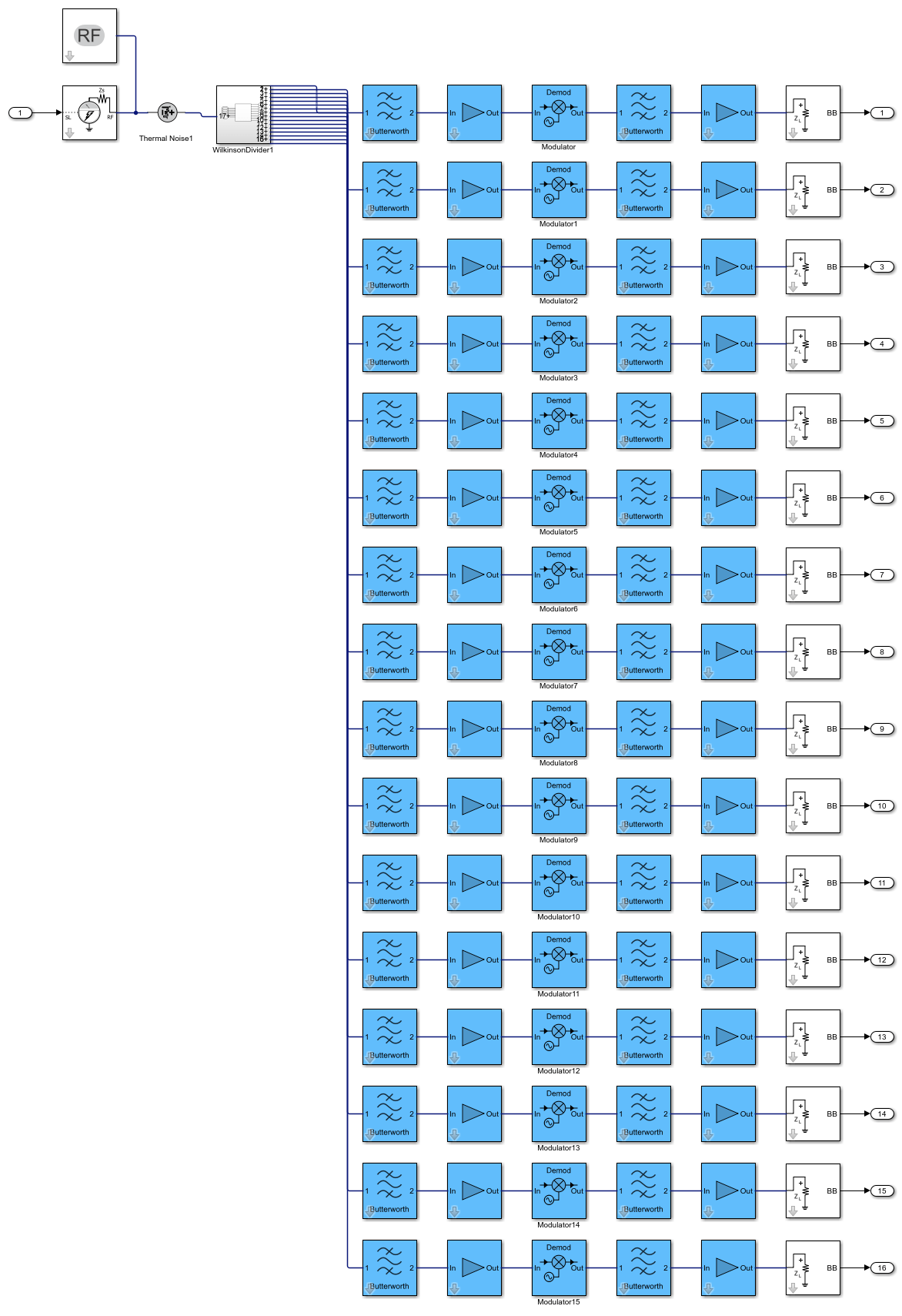Type this command at the MATLAB® command line to create 16 outputs to the SIMO transmitter.

```[out1(1:16).val] = rfs2(in1); ```

Create a fifth-order bandpass RF filter.

```f1 = rffilter('ResponseType','Bandpass','FilterOrder',5,'PassbandFrequency',[4.85 5.15]*1e9); ```

Create an amplifier with the gain of `3` dB, noise figure of `1.53` dB, and OIP3 of `35` dBm.

```a1 = amplifier('Gain',3,'NF',1.53,'OIP3',35); ```

Create an `rfbudget` object using these elements at an input frequency of `5` GHz, an available input power of `-30` dBm, and a bandwidth of `200` MHz.

```rfb = rfbudget([f1 a1],5e9,-30,200e6); ```

Create an RF system using the rfbdget object. Name the model and save the RF Blockset model.

```rfs = rfsystem(rfb,'ModelName','myRFSystem_Model') save_system(rfs); ```
```rfs = rfsystem with properties: ModelName: 'myRFSystem_Model' SampleTime: 6.2500e-10 InputFrequency: 5.0000e+09 OutputFrequency: 5.0000e+09 RFInputs: 1 RFOutputs: 1 Library: 'CircuitEnvelope' ```

Design an RF receiver using the `rfsystem` system object. View the object in the the RF Budget Analyzer app to perform harmonic balance (HB) analysis.

Create fifth- and seventh-order bandpass RF filters.

```f1 = rffilter('ResponseType','Bandpass','FilterOrder',5, ... 'PassbandFrequency',[4.85 5.15]*1e9); f2 = rffilter('ResponseType','Bandpass','FilterOrder',7, ... 'PassbandFrequency',[10 130]*1e6); ```

Create two amplifier objects with `3` dB and `5` dB gain, respectively.

```a1 = amplifier('Gain',3,'NF',1.53,'OIP3',35); a2 = amplifier('Gain',5,'NF',8,'OIP3',37); ```

Create a modulator with a local frequency of `4.93` GHz.

```d = modulator('Gain',0,'NF',4,'OIP3',50,'LO',4.93e9, ... 'ConverterType','Down'); ```

Design an RF receiver with the budget elements at an input frequency of `5` GHz, an available input power of – `30` dBm, and a bandwidth of `10` MHz.

```rfb = rfbudget([f1 a1 d f2 a2],5e9,-30,10e6); ```

Create an RF system for the RF receiver using the `rfbudget` object.

```rfs = rfsystem(rfb); ```

Open an RF Blockset model of the designed RF system using the `open_system` object function.

```open_system(rfs) ```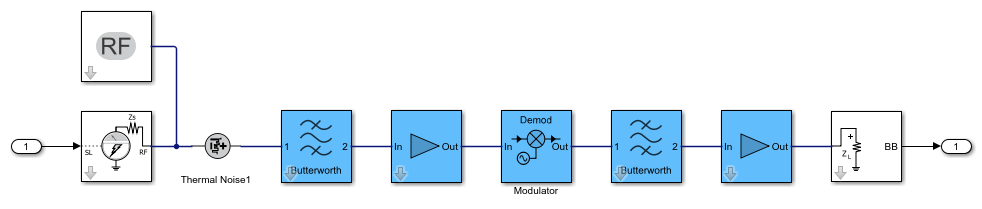Type rfBudgetAnalyzer(rfs) command at the MATLAB® command line to open this RF system in the RF Budget Analyzer app.To conduct HB analysis in the app, click the HB-Analyze button.## Tips

• Create multiple `rfsystem` outputs using this optional syntax:

[`out``(1:n).val`] = `rfs`(`in`)

, where `n` is the number of output chains in a single-input multiple-output (SIMO) transmitter system 'Tx' using the input signal `in`.

## Version History

Introduced in R2021a

expand all